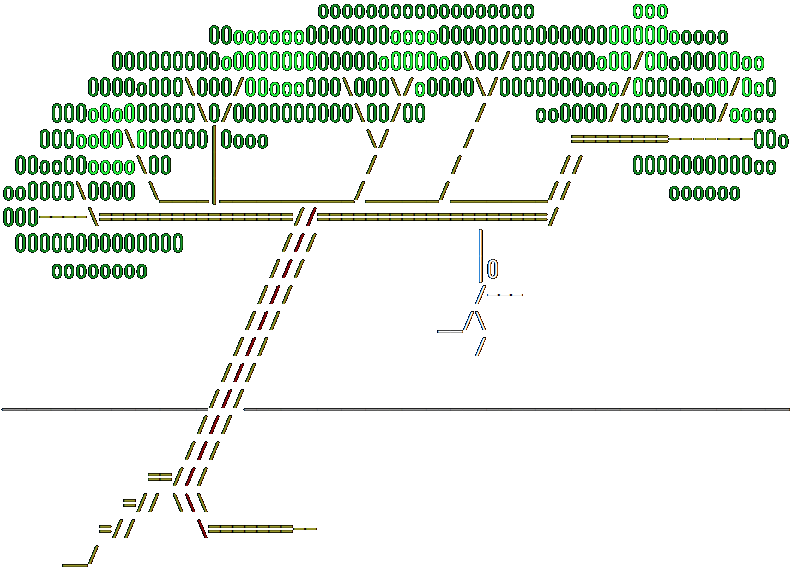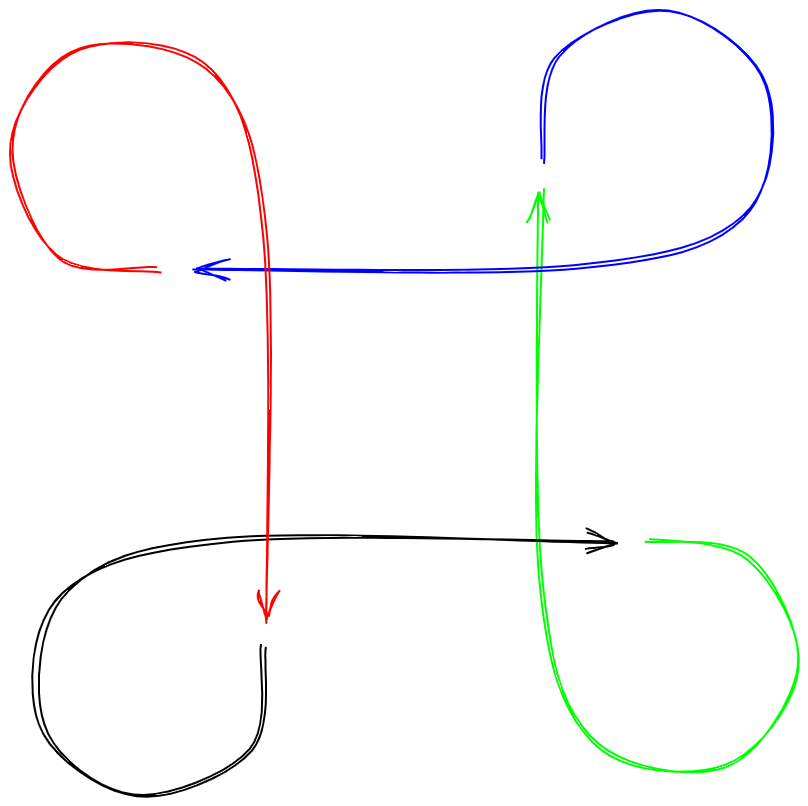# Acyclic Mutually Recursive ClosuresHemlock is a mostly pure functional programming language, and it absolutely prohibits reference cycles in immutable data. To someone steeped in imperative programming idioms this might seem like an insurmountable impediment to getting actual work done. I used to think so, but have in recent years shifted to preferring immutability and acyclicity when at all feasible. And there are some amazing optimization opportunities in the absence of cycles, particularly with regard to parallel garbage collection. That’s a topic for another time; this post is about how Hemlock enforces acyclicity.

It’s easy to create a reference cycle using mutation.

``````type rec t: &t = {
x: uns
&next: option t
}

a = {x=42; next=None}
b = {x=13; next=Some a}
# Tie the knot.
a.next := Some b
``````

How would we create an immutable reference cycle though? We would need some form of forward/mutual declaration. Imagine for a moment that Hemlock allows the following (it does not).

``````type rec t: t = {
x: uns
next: t
}

# Poof, a knot.
a = {x=42; next=b}
also b = {x=13; next=a}
``````

Disaster is averted simply by omitting such constructs from Hemlock. But Hemlock does provide one seemingly dangerous construct: mutual recursion.

``````rec odd x =
match x > 0 with
| false -> "odd"
| true -> even (x - 1)
also even x =
match x > 0 with
| false -> "even"
| true -> odd (x - 1)
f = even
f 42 # -> "even"
f 13 # -> "odd"
``````

At the time the `odd` closure is created, `even` isn’t yet defined. A straightforward implementation would just mutate `odd` once `even` exists. Or the implementation could treat `even` as a “free variable” in the `odd` closure. Let’s look at a manual implementation of this that uses partial application to allocate closures and see why it’s a form of cycle-inducing mutation.

``````type odd_closure: &odd_closure = {
&even: uns -> string
}

odd_func {even} x =
match x > 0 with
| false -> "odd"
| true -> even (x - 1)

type even_closure: &even_closure = {
&odd: uns -> string
}

even_func {odd} x =
match x > 0 with
| false -> "even"
| true -> odd (x - 1)

dummy_func _x =
""

even = {odd=dummy_func}
even.odd := odd_func even
odd = {even=dummy_func}
odd.even := even_func odd
``````

Okay, let’s do something sightly more clever that has a long enough name even the German language would be moderately proud: defunctionalization.

``````type selector =
| Odd of uns
| Even of uns

rec odd_even selector =
match selector with
| Odd x ->
match x > 0 with
| false -> "odd"
| true -> odd_even (Even (x - 1))
| Even x ->
match x > 0 with
| false -> "even"
| true -> odd_even (Odd (x - 1))

odd x =
odd_even (Odd x)

even x =
odd_even (Even x)
``````

It is possible to generate machine code that is incrementally faster than variant dispatch, but this captures the essence of the approach, and it can always convert mutually recursive functions to a form that avoids reference cycles. Thank goodness, Hemlock programs can use mutual recursion without restriction!